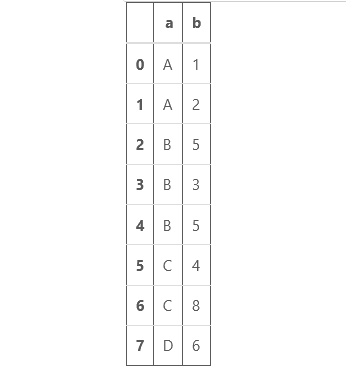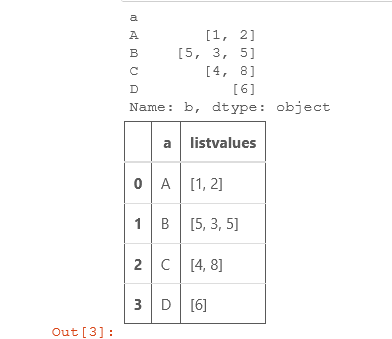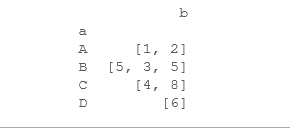Related Articles

# How to List values for each Pandas group?

• Last Updated : 20 Aug, 2020

In this article, we’ll see how we can display all the values of each group in which a dataframe is divided. The dataframe is first divided into groups using the DataFrame.groupby() method. Then we modify it such that each group contains the values in a list.

First, Let’s create a Dataframe:

## Python3

 `# import pandas library``import` `pandas as pd`` ` `# create a dataframe``df ``=` `pd.DataFrame({``'a'``: [``'A'``, ``'A'``, ``'B'``,``                          ``'B'``, ``'B'``, ``'C'``,``                          ``'C'``, ``'D'``], ``                    ``'b'``: [``1``, ``2``, ``5``,``                          ``3``, ``5``, ``4``,``                          ``8``, ``6``]})``# show the dataframe                  ``df`

Output:Method 1: Using DataFrame.groupby() and Series.apply() together.
Example: We’ll create lists of all values of each group and store it in new column called “listvalues”.

## Python3

 `# import pandas library``import` `pandas as pd`` ` `# create a dataframe``df ``=` `pd.DataFrame({``'a'``: [``'A'``, ``'A'``, ``'B'``,``                          ``'B'``, ``'B'``, ``'C'``,``                          ``'C'``, ``'D'``], ``                    ``'b'``: [``1``, ``2``, ``5``,``                          ``3``, ``5``, ``4``,``                          ``8``, ``6``]})``                  ` `# convert values of each group``# into a list``groups ``=` `df.groupby(``'a'``)[``'b'``].``apply``(``list``)`` ` `print``(groups)`` ` `# groups store in a new ``# column called listvalues``df1 ``=` `groups.reset_index(name ``                         ``=` `'listvalues'``)``# show the dataframe``df1`

Output:Method 2: Using DataFrame.groupby() and Series.agg().

Example: We use the lambda function inside the Series.agg() to convert the all values of a group to a list.

## Python3

 `# import pandas library``import` `pandas as pd`` ` `# create a dataframe``df ``=` `pd.DataFrame( {``'a'``: [``'A'``, ``'A'``, ``'B'``,``                         ``'B'``, ``'B'``, ``'C'``, ``                         ``'C'``, ``'D'``], ``                    ``'b'``: [``1``, ``2``, ``5``, ``                         ``3``, ``5``, ``4``,``                         ``8``, ``6``]}``                 ``)``# convert values of each group``# into a list``groups ``=` `df.groupby(``'a'``).agg(``lambda``                             ``x: ``list``(x))`` ` `print``(groups)`

Output:Attention geek! Strengthen your foundations with the Python Programming Foundation Course and learn the basics.

To begin with, your interview preparations Enhance your Data Structures concepts with the Python DS Course. And to begin with your Machine Learning Journey, join the Machine Learning – Basic Level Course

My Personal Notes arrow_drop_up